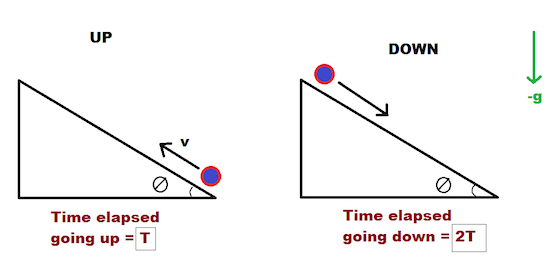# Once Up, Twice DownA point-mass (particle) is shot up an inclined plane that makes an angle $\theta$ with the ground. It slides up the incline to a certain distance, and then slides all the way back down. However, due to friction, the time it takes for the particle to slide from the maximum distance it reaches up the incline back to the bottom is twice the time it takes for it to go to the maximum distance up the incline from the bottom.

The coefficient of friction $\mu$ between the particle and the incline can be written as $\frac{a}{b}\tan\theta$, where $a$ and $b$ are co-prime positive integers.

What is the value of $a + b$?

×